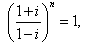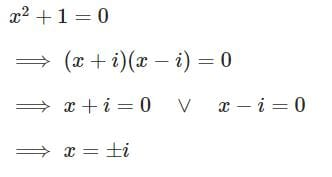Test Description

## 10 Questions MCQ Test Mathematics (Maths) Class 11 | Test: Quadratic Equations

Test: Quadratic Equations for JEE 2023 is part of Mathematics (Maths) Class 11 preparation. The Test: Quadratic Equations questions and answers have been prepared according to the JEE exam syllabus.The Test: Quadratic Equations MCQs are made for JEE 2023 Exam. Find important definitions, questions, notes, meanings, examples, exercises, MCQs and online tests for Test: Quadratic Equations below.
Solutions of Test: Quadratic Equations questions in English are available as part of our Mathematics (Maths) Class 11 for JEE & Test: Quadratic Equations solutions in Hindi for Mathematics (Maths) Class 11 course. Download more important topics, notes, lectures and mock test series for JEE Exam by signing up for free. Attempt Test: Quadratic Equations | 10 questions in 10 minutes | Mock test for JEE preparation | Free important questions MCQ to study Mathematics (Maths) Class 11 for JEE Exam | Download free PDF with solutions
 1 Crore+ students have signed up on EduRev. Have you?
Test: Quadratic Equations - Question 1

### Solve the quadratic equation x2 – ix + 6 = 0

Detailed Solution for Test: Quadratic Equations - Question 1

x2 - ix + 6 = 0
x2 - 3ix + 2ix - 6i2 = 0    { i2 = -1}
x(x-3i) + 2i(x-3i) = 0
(x+2i) (x-3i) = 0
x = -2i, 3i

Test: Quadratic Equations - Question 2

###so the least integral value of n is

Detailed Solution for Test: Quadratic Equations - Question 2

{(1 + i)/(1 - i)}n = 1
multiply (1 + i) numerator as well as denominator .
{(1 + i)(1 + i)/(1 - i)(1 + i)}n = 1
{(1 + i)²/(1² - (i)²)}n = 1
{(1 + i² +2i)/2 }n = 1
{(2i)/2}n = 1
{i}n = 1
we know, i4n = 1 where , n is an integer.
so, n = 4n where n is an integers
e.g n = 4 { because least positive integer 1 }
hence, n = 4

Test: Quadratic Equations - Question 3

### Solve the quadratic equation ix2 – 3x – 2i = 0

Detailed Solution for Test: Quadratic Equations - Question 3

x2−3x−2i=0,

or  x2 + 3ix − 2=0      (divided by i)
x= −3i ± [−9 + 4.1.2]1/2/2.1
= −3i ±  (−1)1/2/2
​= (−3i ± i)/2
=> x=−i or x=−2i

Test: Quadratic Equations - Question 4

Find the roots of the quadratic equation: x2 + 2x - 15 = 0?

Detailed Solution for Test: Quadratic Equations - Question 4

x2 + 5x - 3x - 15 = 0
x(x + 5) - 3(x + 5) = 0
(x - 3)(x + 5) = 0
⇒ x = 3 or x = -5.

Test: Quadratic Equations - Question 5

Solve the quadratic equation x2 +1 = 0

Detailed Solution for Test: Quadratic Equations - Question 5Test: Quadratic Equations - Question 6

The solution of the quadratic equation: 2x2 + 3ix + 2 = 0

Detailed Solution for Test: Quadratic Equations - Question 6

2x2 + 3ix + 2 = 0
we know, x = (-b ± √b2 - 4ac)/2a
x =  [-3i ± √(3i)2 - 4x2x2]/2x2
= -3i ± √-25/4
= i(-3±5)/4
x = i/2, -2i

Test: Quadratic Equations - Question 7

The solution of the quadratic equation : 2x2 – 4x + 3 = 0

Detailed Solution for Test: Quadratic Equations - Question 7

2x2 - 4x + 3 = 0
x = [-(-4) +- (√16-24)]/2(2)
x = (4 +- i√8)/4
x = (4 +- 2i√2)/(2 * √2 * √2)
x = 2(2 +- i√2)/(2 * √2 * √2)
x = 1 +- i/√2

Test: Quadratic Equations - Question 8

If one of the root of a quadratic equation with rational coefficients is rational, then other root must be

Detailed Solution for Test: Quadratic Equations - Question 8

Also, αβ = r/p, which is also rational. α + β = (a+√b) + (a-√b) = 2a, a rational number and, αβ = (a+√b)(a-√b) = a² - b, a rational number. So, the other root of a quadratic equation having the one root as (a+√b) is (a-√b), where a and b are rational numbers.

Test: Quadratic Equations - Question 9

If the sum of three consecutive terms of an increasing A.P. is 51 and the product of the first and third of these terms is 273, then the third term is

Detailed Solution for Test: Quadratic Equations - Question 9

Let these three terms are, a−d,aanda+d.
Then,
a−d+a+a+d=51⇒3a=51⇒a=17
Now, (a−d)(a+d)=273
⇒(17−d)(17+d)=273
⇒289−d2=273⇒d2=16
⇒d=4 (As d can not be negative.)
So, third term will be, 17+4=21.

Test: Quadratic Equations - Question 10

Solve the quadratic equation 9x2 + 16 = 0

Detailed Solution for Test: Quadratic Equations - Question 10

9x2 + 16 = 0
9x2 = -16
x2 = -16/9
x = ± 4/3 i

## Mathematics (Maths) Class 11

156 videos|176 docs|132 tests(Scan QR code)